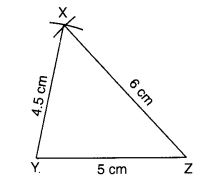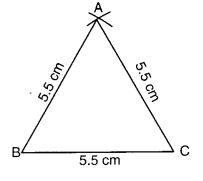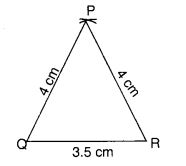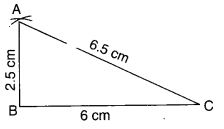NCERT Solutions for Class 7 Maths Chapter 10 Practical Geometry Ex 10.2 are part of NCERT Solutions for Class 7 Maths. Here we have given NCERT Solutions for Class 7 Maths Chapter 10 Practical Geometry Ex 10.2.

 Board CBSE Textbook NCERT Class Class 7 Subject Maths Chapter Chapter 10 Chapter Name Practical Geometry Exercise Ex 10.2 Number of Questions Solved 4 Category NCERT Solutions

## NCERT Solutions for Class 7 Maths Chapter 10 Practical Geometry Ex 10.2

Question 1.
Construct A XYZ in which XY = 4.5 cm, YZ = 5 cm and, ZX = 6 cm.
Solution:
Steps of Construction

1. Draw a line segment YZ of length 5 cm.2. With Y as centre, draw an arc of radius 4.5 cm.
3. With Z as centre, draw an arc of radius 6 cm,
4. Mark the point of intersection of arcs as X.
5. Join XY and XZ. ∆ XYZ is now ready.

Question 2.
Construct an equilateral triangle of side 5.5 cm.
Solution:
Steps of Construction:

1. Draw a line segment BC of length 5.5 cm.
2. With B as centre, draw an arc of radius 5.5 cm.3. With C as centre, draw an arc of radius 5.5 cm.
4. Mark the point of intersection of arcs as A.
5. Join AB and AC. Equilateral ∆ ABC is now ready.

Question 3.
Draw ∆ PQR with PQ = 4 cm, QR =3.5 cm and PR = 4 cm. What type of triangle is this?
Solution:
Steps of Construction:

1. Draw a line segment QR of length 3.5 cm.
2. With Q as centre, draw an arc of radius 4 cm.
3. With R as centre, draw an arc of radius 4 cm.4. Mark the point of intersection of arcs as P.
5. Join PQ and PR.

∆ PQR is now ready,
∵ PQ = PR
∴ ∆ PQR is isosceles.

Question 4.
Construct ∆ ABC such that AB = 2.5 cm, BC = 6 cm and AC = 6.5 cm. Measure ∠B.
Solution:
Steps of Construction

1. Draw a line segment BC of length 6 cm.2. With B as centre, draw an arc of radius 2.5 cm.
3. With C as centre, draw an arc of a radius of 6.5 cm.
4. Mark the point of intersection of arcs as A.
5. Join AB and AC.
6. ∆ ABC is now ready. On measurement, ∠B = 90°.

We hope the NCERT Solutions for Class 7 Maths Chapter 10 Practical Geometry Ex 10.2 help you. If you have any query regarding NCERT Solutions for Class 7 Maths Chapter 10 Practical Geometry EX 10.2, drop a comment below and we will get back to you at the earliest.